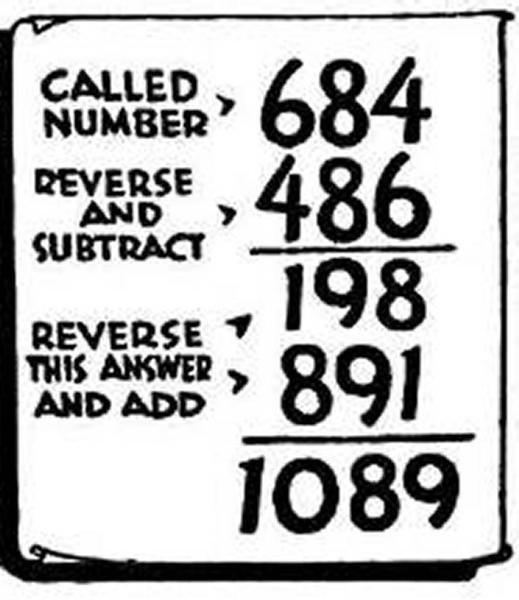# Math curiosities (2)

in #math2 years ago

### 1089

##### If you pick one three-digit numbers, you can "end" with the 1089 number.### Try yourself

example: 275

##### Reverse digits and subtract the smaller number from the larger one

example: 572-275=297

##### To this result add the number produced by reversing digits of result number

example: 297+792=1089

##### 🎩🤪
Sort: